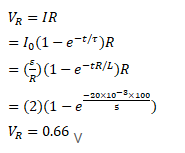# An inductor of resistance 5.0 H,

Question:

An inductor of resistance $5.0 \mathrm{H}$, having a negligible resistance, is connected in series with a $100 \Omega$ resistor and a battery of emf $2.0 \mathrm{~V}$. Find the potential difference across the resistor $20 \mathrm{~ms}$ after the circuit is switched on.

Solution: